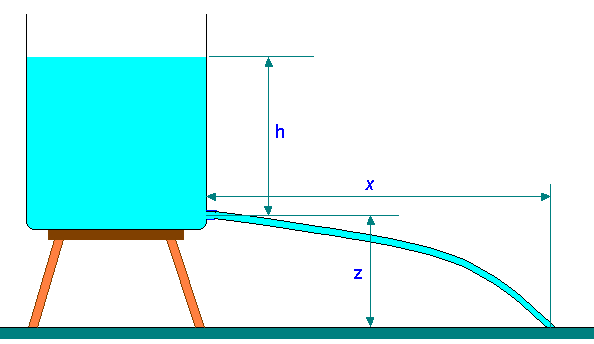### Trajectory of Jet issued from an orifice at the side of a tank opened to atmosphere

Home -> Lecture Notes -> Fluid Mechanics -> Unit-IIAt the tip of the opening:

The horizontal component of jet velocity Vx = (2gh)0.5 = dx/dt

And the vertical component Vz = 0

One the jet is left the orifice, it is acted upon by gravitational forces. This makes the vertical component of velocity to equal ‘-gt’.

i.e., Vz = -gt = dz/dt

The horizontal and vertical distances covered in time ‘t’ are, obtained from integrating the above equations.

x = (2gh)0.5 t

and z = -gt2/2

And elimination of ‘t’ can be done as,

z = -g [x2/(2gh)] / 2

i.e,

z = - x2/(4h)

Let us take downward direction as positive z. Then

x = 2 (hz)0.5